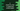# C program to check if a character is uppercase using isupper## C program to check if a character is uppercase using isupper:

In this post, we will learn how to check if a character is uppercase character or not using isupper function. This function is defined in the ctype.h header file. In this post, we will learn how to use this function with its definition and examples.

### Definition of isupper:

The isupper function is defined as like below:

``int isupper(int c)``

It takes the character to check as its argument. The argument type is int but we can pass a character. It converts that character to integer i.e. it takes the ASCII value for that character.

It returns an integer value defining if the character is a uppercase character or not. If yes, it returns a non-zero value, else it returns 0.

This method is defined in the ctype.h header file.

### Example of isupper function:

Let me show it with an example:

``````#include <stdio.h>
#include <ctype.h>

int main()
{
char charArray[] = {'a', 'A', 'e', '1', '&', '(', 'X'};

for (int i = 0; i < 7; i++)
{
printf("isupper(%c) => %d\n", charArray[i], isupper(charArray[i]));
}
return 0;
}``````

In this program, we have initialized an array of characters charArray. The for loop iterates through the characters of the array one by one and prints the value of isupper.

If you run this program, it will print:

``````isupper(a) => 0
isupper(A) => 1
isupper(e) => 0
isupper(1) => 0
isupper(&) => 0
isupper(() => 0
isupper(X) => 1``````

As you can see here, for A and X, it returns 1 and for other characters it returns 0.

### isupper with if-else:

Since isupper returns a non-zero value or 0, we can use it with if-else.

``````#include <stdio.h>
#include <ctype.h>

int main()
{
char charArray[] = {'a', 'A', 'e', '1', '&', '(', 'X'};

for (int i = 0; i < 7; i++)
{
if (isupper(charArray[i]))
{
printf("'%c' is in uppercase\n", charArray[i]);
}
}
return 0;
}``````

This program is using the same array we used in the previous example. It checks the return value of isupper and if it is 1, it prints the character.

So, it will print only the uppercase characters:

``````'A' is in uppercase
'X' is in uppercase``````

### isupper with user input characters:

Let’s use isupper with user input characters. The below program will take a character as the input from the user and prints if it is uppercase or lowercase:

``````#include <stdio.h>
#include <ctype.h>

int main()
{
char c;

printf("Enter a character: ");
scanf("%c", &c);

if (isupper(c))
{
printf("%c is in uppercase\n",c);
}
else
{
printf("%c is in lowercase\n",c);
}
return 0;
}``````

This program takes a character as an input from the user. It uses isupper to check if the character is in upper-case or not. Based on the result, it prints a message.

It will give output as like below:

``````Enter a character: c
c is in lowercase

Enter a character: C
C is in uppercase``````